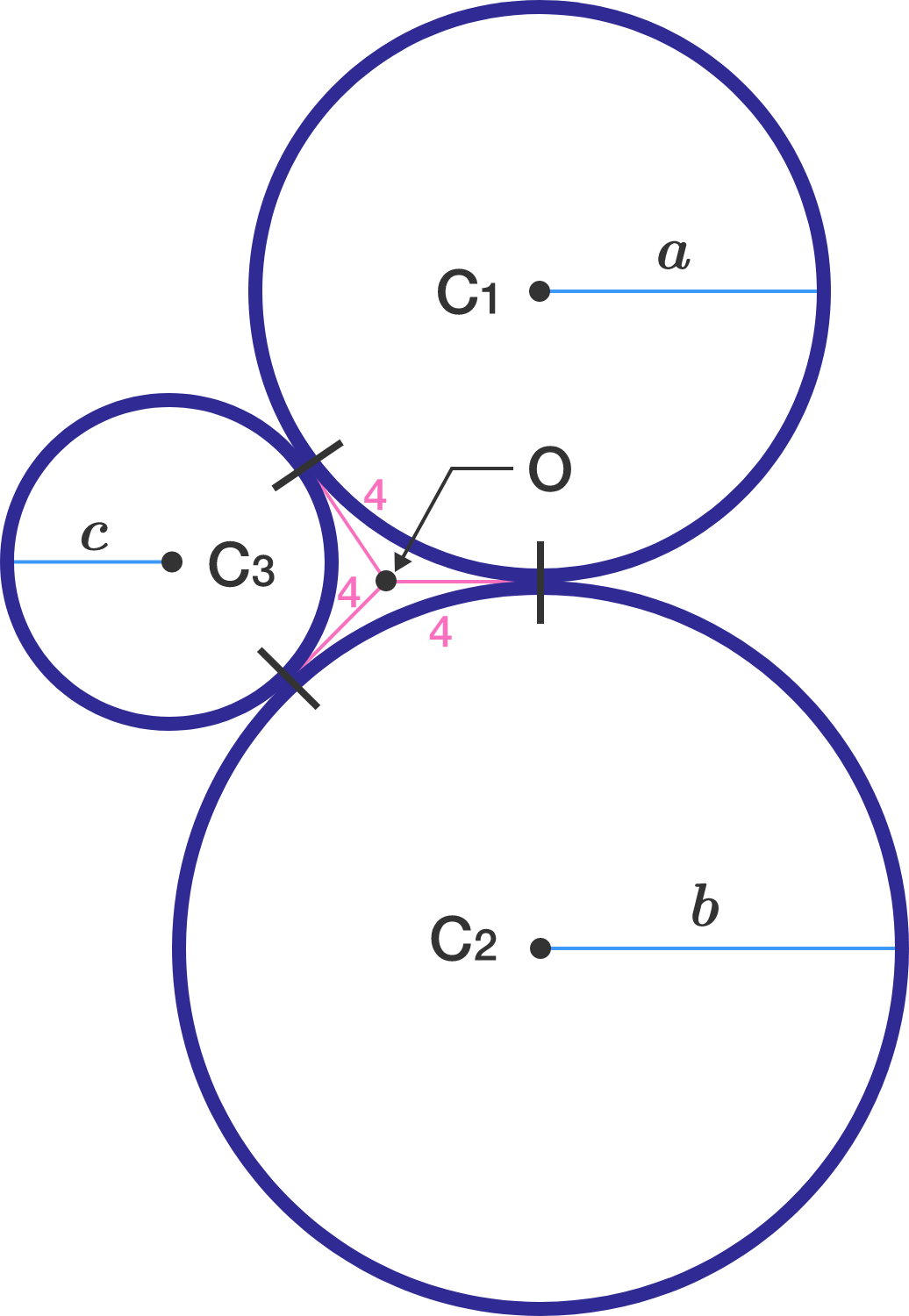# Power of three!

Geometry Level 4Three circles $C_1, C_2, C_3$ of radii $a , b, c$, respectively, touch each other externally at three different points, as shown in the figure above.

If their points of contact are all 4 units away from the radical center $O$ of the three circles, then evaluate $\dfrac{abc}{a+b+c}.$

×